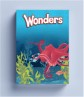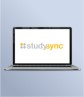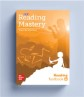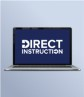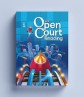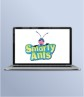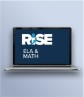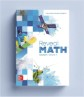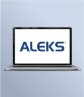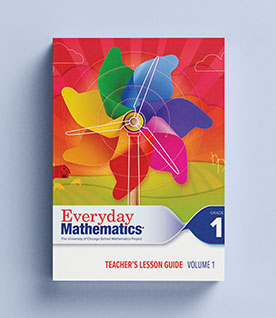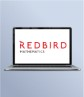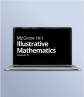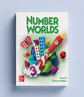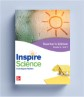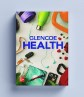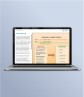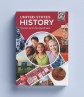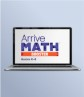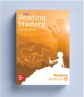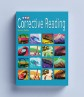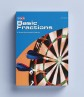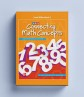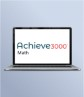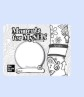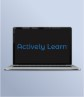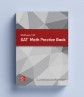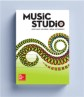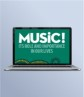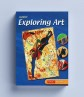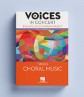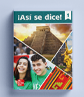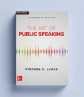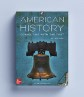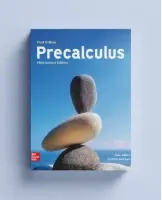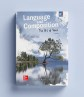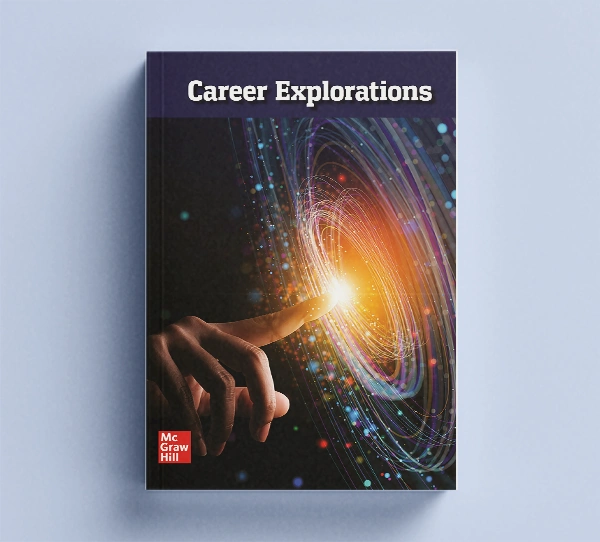## Our Principles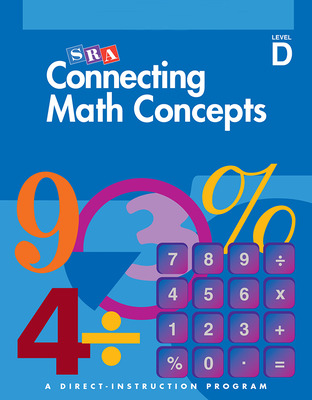# Connecting Math Concepts 2003

Connecting Math Concepts introduces ideas gradually and teaches students the connections between concepts. Focusing on the big ideas of mathematics, Connecting Math Concepts teaches explicit strategies that enable students to master difficult ideas such as ratios, proportions, probability, functions, and data analysis. Detailed explanations and guided practice move students toward independent work, ensuring that they gain success and confidence as mathematical thinkers.

## Program Details

This highly successful program makes it easier than ever to teach students to become capable problem solvers, able to think and communicate mathematically. Through a unique pedagogical approach, Connecting Math Concepts provides a thorough understanding of basic skills, shows how mathematical concepts are linked together, and builds sophisticated problem-solving strategies.

With Connecting Math Concepts, all concepts are learned and assimilated through use. New concepts are presented incrementally and extended into subsequent lessons. Students move forward in small steps, learning and applying many topics in each lesson. Students learn in less time, remember more, and develop a depth of understanding needed for advanced mathematics.

Features:

• Teaches every student to discover and use math patterns
• Ideas are introduced at a carefully controlled rate with systematic, continuous review.
• Takes the mystery out of story problems!

Student Materials:

Student Workbook (Level A-E)

• Provides challenging application activities
• Workbooks give each student extra practice so students master the basics as they expand their understanding of mathematics
• Correspond to each lesson so students get the most out of each concept

Student Hardcover Textbook (Level D-F)

• Provide challenging application activities
• Clear and concise directions for each activity
• Independent work perfect for seat work or take home activities
• Mathematical rules and examples for easy recall

Teacher Materials:

Teacher Materials Packages include 1 or 2 Presentation Books, Teacher's Guide, and Answer Key

• Presentation Books provide fully scripted lesson plans that make teaching efficient and highly effective
• Feature instructional strategies, teaching tips, and a Scope and Sequence in the Teacher Guide
• Clearly labeled answer keys, making it easy to check independent work

• Feature instructional strategies, teaching tips, and a Scope and Sequence, plus a reproducible Placement Test

• Clearly labeled answer keys, making it easy to check independent work

Lesson Sampler

• Includes sample lessons, reproducible placement tests, and scope and sequence charts for all levels of the program

Math Facts Blackline Masters (Level A-D)

• Provide extra worksheets for students who require extra practice in math facts
• Test pages for teachers to assess student's mastery of the facts

Independent Worksheets Blackline Masters (Levels A-F)

• Additional practice for skill and mastery maintenance
• Ideal for homework activities or for independent use in the classroom